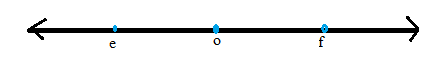Chapter A.3, Problem 2EElementary Geometry For College St...

7th Edition
Alexander + 2 others
ISBN: 9781337614085

Solutions

Chapter
SectionElementary Geometry For College St...

7th Edition
Alexander + 2 others
ISBN: 9781337614085
Textbook Problem

Using the number line shown, write two inequalities that relate the values of e and f.To determine

To write:

The two inequalities that relates the values of e and f by using the following number line,Explanation

Consider the following number line,

The above number line shows that e is left of o and similarly f is right of o.

That is e is before number of o.

Therefore, e<o

Similarly, f is after number of o

Therefore, f<

Still sussing out bartleby?

Check out a sample textbook solution.

See a sample solution

The Solution to Your Study Problems

Bartleby provides explanations to thousands of textbook problems written by our experts, many with advanced degrees!

Get Started

Factor each expression in Problems 7-18 as a product of binomials. 13.

Mathematical Applications for the Management, Life, and Social Sciences

A sample of n = 9 scores has X = 108. What is the sample mean?

Essentials of Statistics for The Behavioral Sciences (MindTap Course List)

Define the alpha level and the critical region for a hypothesis test.

Statistics for The Behavioral Sciences (MindTap Course List)

In Exercises 47-52, find and simplify f(a+h)f(a)h(h0) for each function. 50. f(x) = 2x3 x2 + 1

Applied Calculus for the Managerial, Life, and Social Sciences: A Brief Approach

The polar form for the graph at the right is:

Study Guide for Stewart's Single Variable Calculus: Early Transcendentals, 8th